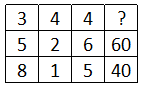Question 1

# In the following question, select the number which can be placed at the sign of question mark (?) from the given alternatives.Solution

In each row, the number at the end is equal to the product of first three numbers.

Eg :- $$5\times2\times6=60$$

and $$8\times1\times5=40$$

Similarly, $$3\times4\times4=48$$

=> Ans - (B)